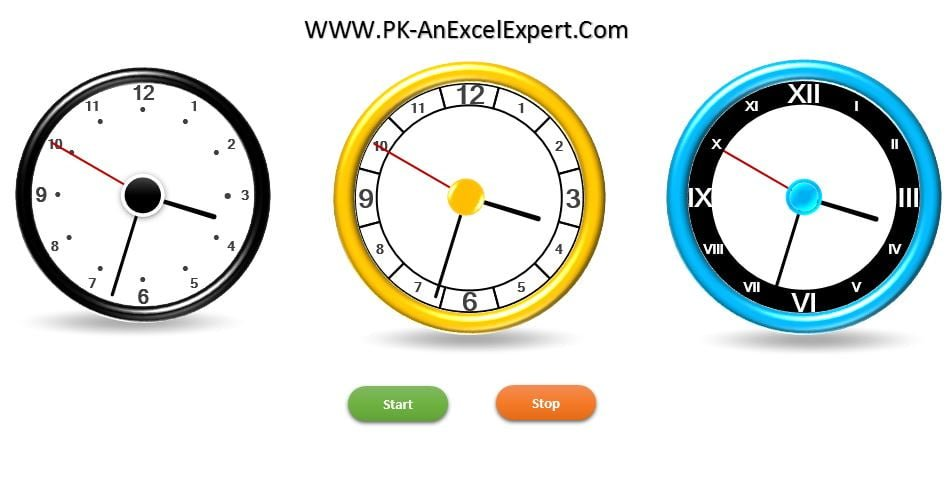Home>Blogs>Charts and Visualization>Analog Clock in Excel (Pie Chart + Doughnut Chart)

# Analog Clock in Excel (Pie Chart + Doughnut Chart)

After creating the Digital Clock, we have created a beautiful Analog Clock. This stunning Analog Clock we have developed using Pie Chart and Doughnut Chart. To calculate the formula automatically, we have used below given code.

```Option Explicit

Sub Start_Clock()

Dim sh As Worksheet
Set sh = ActiveSheet

sh.Range("N1").Value = "Start"

x:
VBA.DoEvents
If sh.Range("N1").Value = "Stop" Then Exit Sub
Application.Calculate
GoTo x

End Sub

Sub Stop_Clock()

Dim sh As Worksheet
Set sh = ActiveSheet

sh.Range("N1").Value = "Stop"

End Sub```

Below is the image of stunning Analog clocks-